# 高级算法与工程

Machine Learning - Android Ng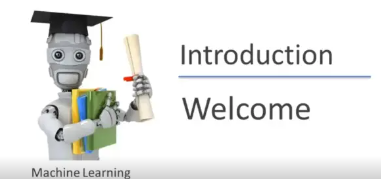## 4.1. 评价 ml 系统

• 计算测试误差的函数就是减去正则化项的损失函数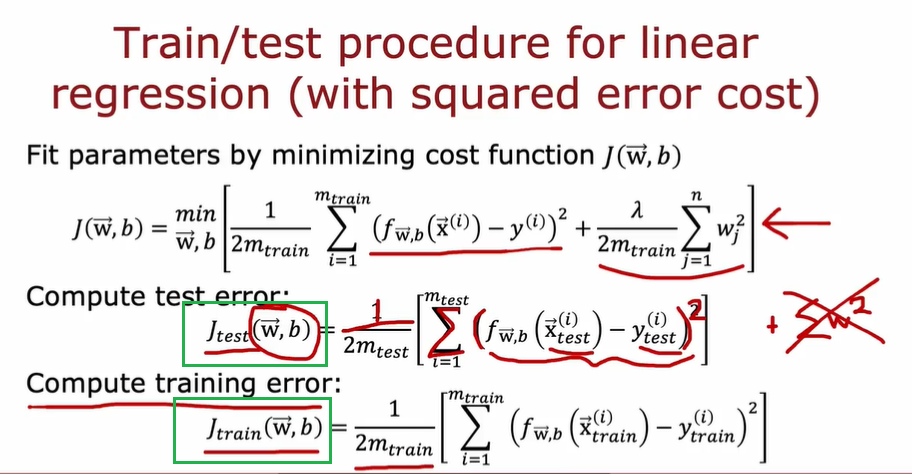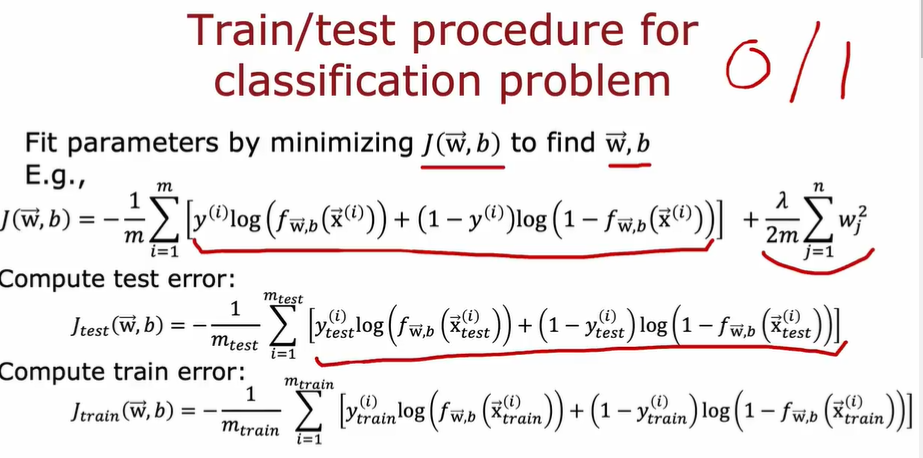### 4.1.1. 选择模型

• 把数据集分为训练集、测试集和交叉验证集
• 中心思想：更加公平（不是更加准确）
• 先选择交叉验证误差最小的模型
• 可以使用测试集误差来代表泛化误差，评估模型的泛化误差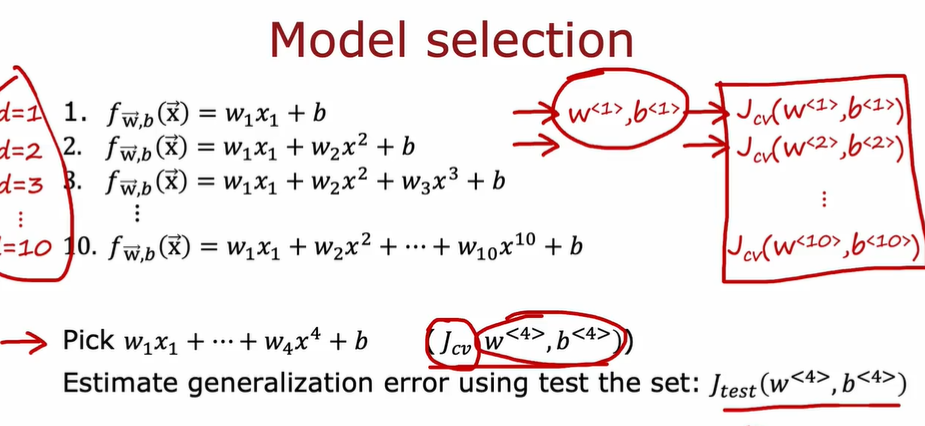### 4.1.2. 诊断模型

• 如何决定第二步做什么，以提高学习的效果

• 方法：观察算法的偏差和方差（Bias and Variance）

• $J_{train}$很高时，往往意味着 Bias 高（欠拟合）

• $J_{cv}$高时，且$J_{cv}$比$J_{train}$高很多时，往往意味着 Variance 高（过拟合）

$J_{cv}$和$J_{train}$都比较低时，是比较理想的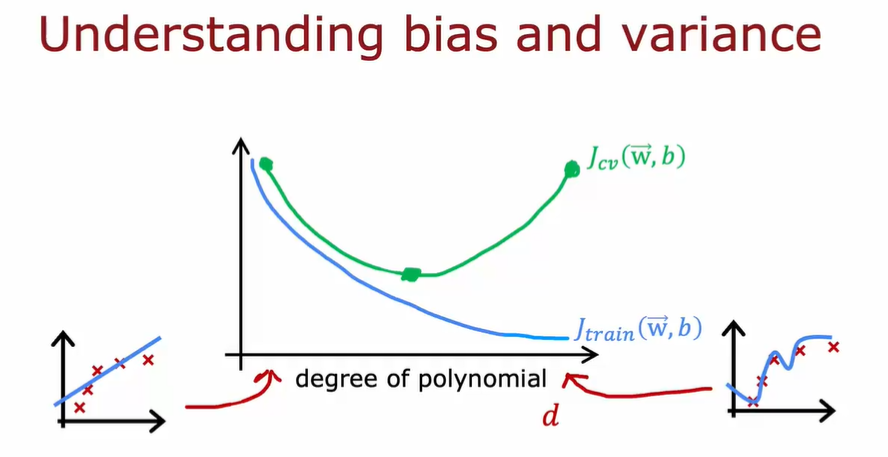### 4.1.4. 选择正则化参数 λ

λ ~ w 的值 ~ J

• 测试过程如下，选择$J_{cv}$最小的模型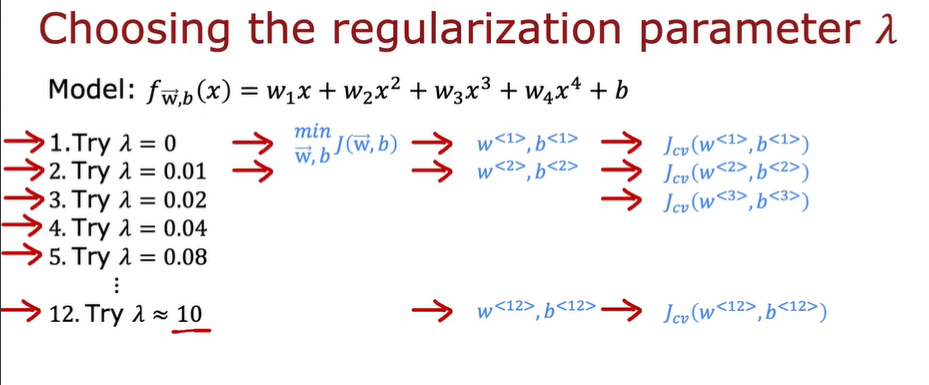• λ 和$J$的关系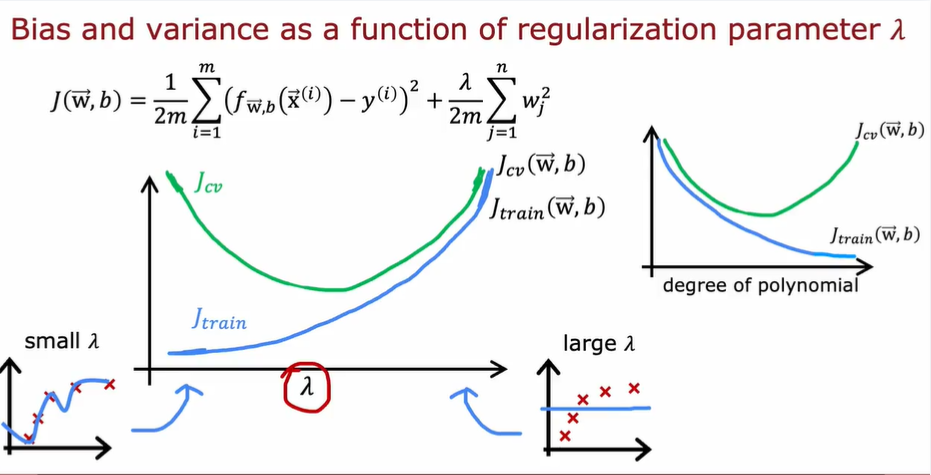### 4.1.4. 如何评价模型错误率

• 与其直接看误差，不如以人类水平为基准进行评判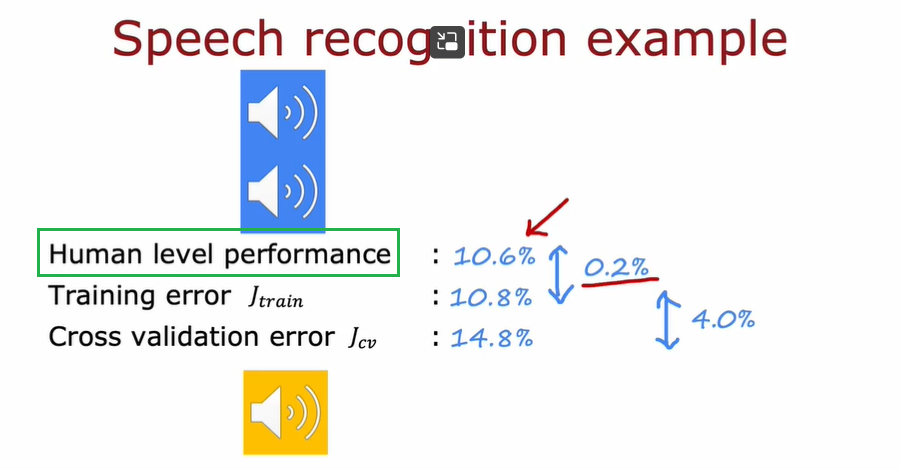#### 4.1.4.1. 性能基准

• 人类水平（常用于非结构化数据，如图像、语音、文本）
• 竞争对手的算法表现
• 经验

• 基准线水平
• 训练误差($J_{train}$)
• 交叉验证误差($J_{cv}$)
• 前两者之间的差 indicates bias，后两者之间的差 indicates variance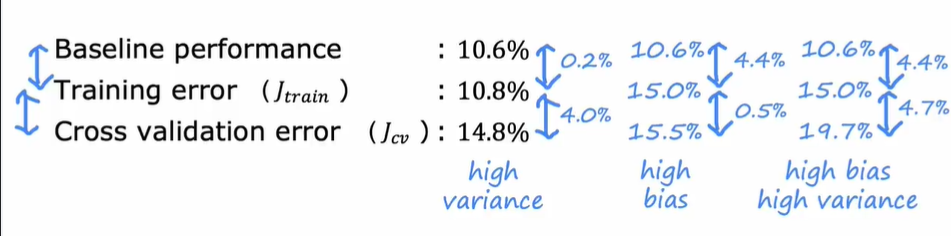### 4.1.5. 学习曲线

#### 4.1.5.2. 神经网络应用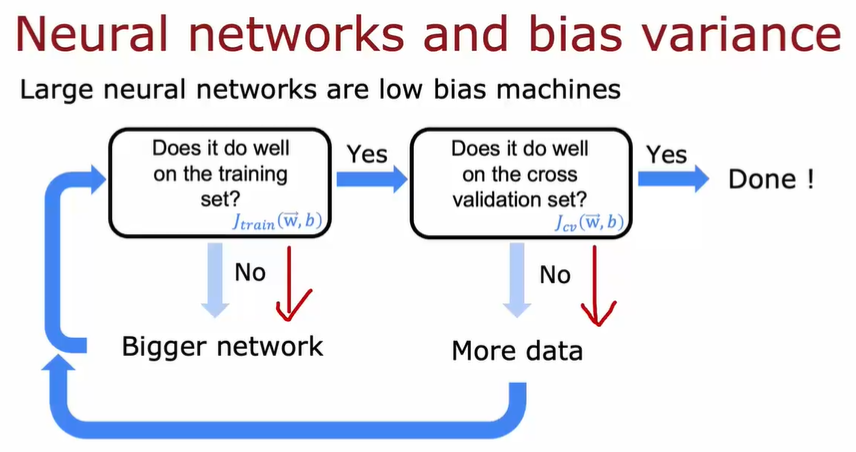• tf 代码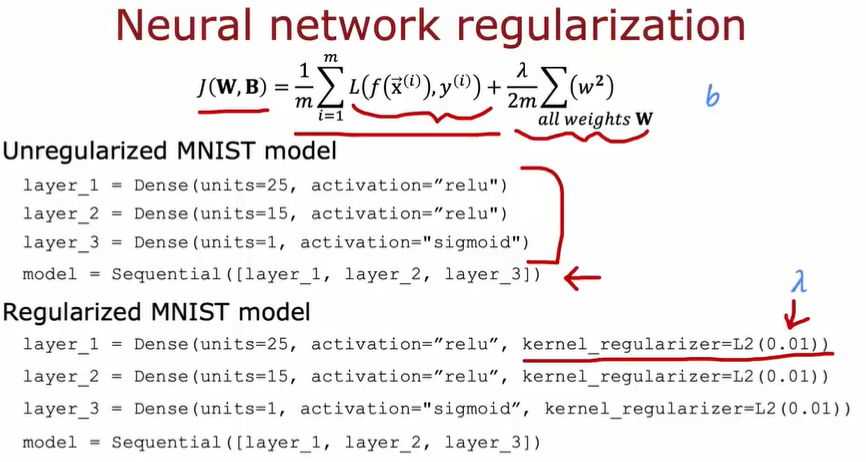#### 4.1.5 误差分析

• 人工检查 100 个数据集，并将它们按照共同特征分类
• 然后进行一些针对性补丁，比如添加一些更加具有针对性的数据集

## 4.2. 数据增强

• Data argumentation
• 通过已有的训练集来进行新的训练：比如把训练集 A image 进行一些变化，然后重新进行训练（人为的增大训练集）
• 注意：对数据所作的改变和扭曲，应该是测试集中噪声或变形的代表
• 随机无意义的噪声和扭曲是没有意义的
• 广泛的应用于图像和音频中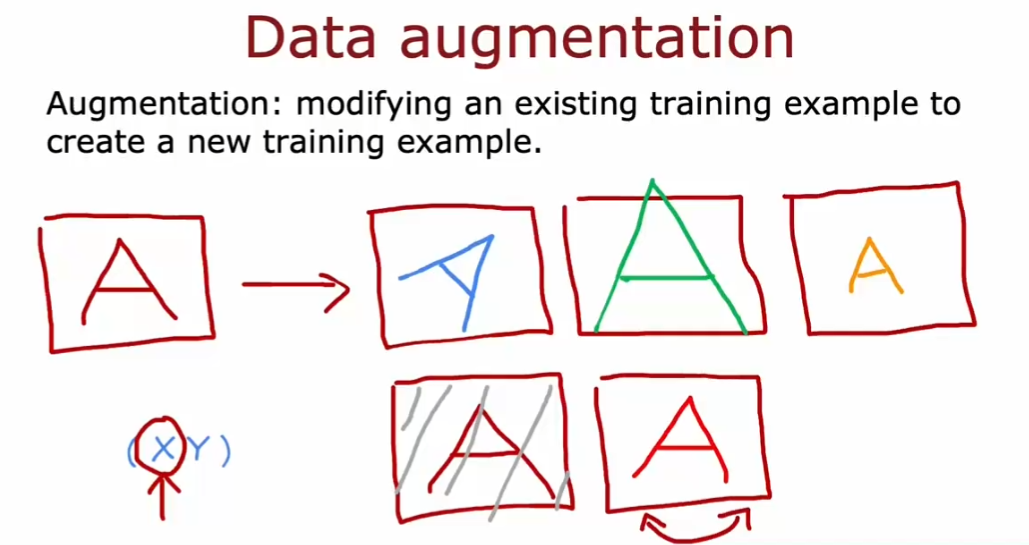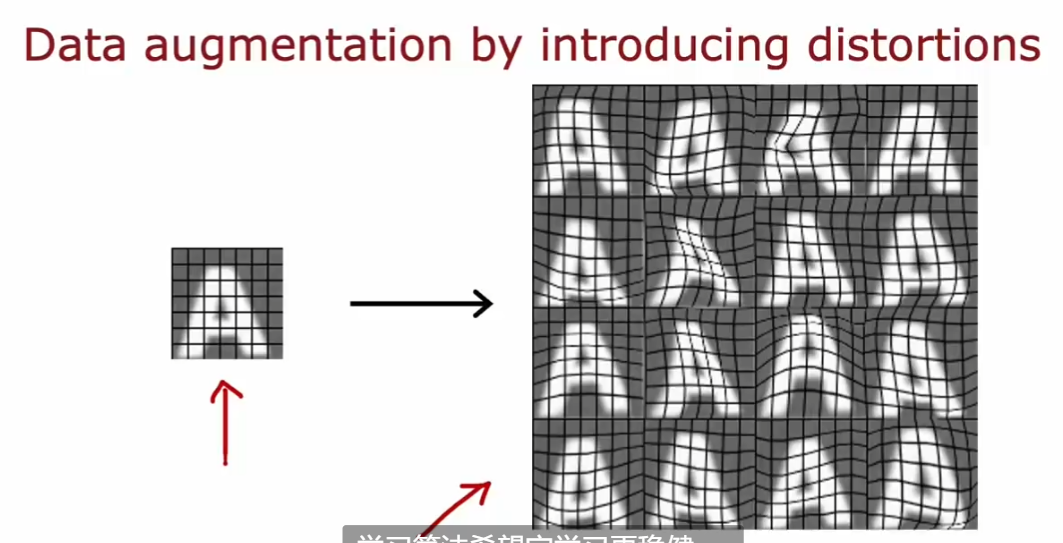## 4.3. 迁移学习

• 可以用来自不同任务的数据完成学习
• 应对数据少或数据难以获取的情况

• 只训练 output 层的参数
• 训练所有参数。但是以 copy 的神经网络参数作为初始值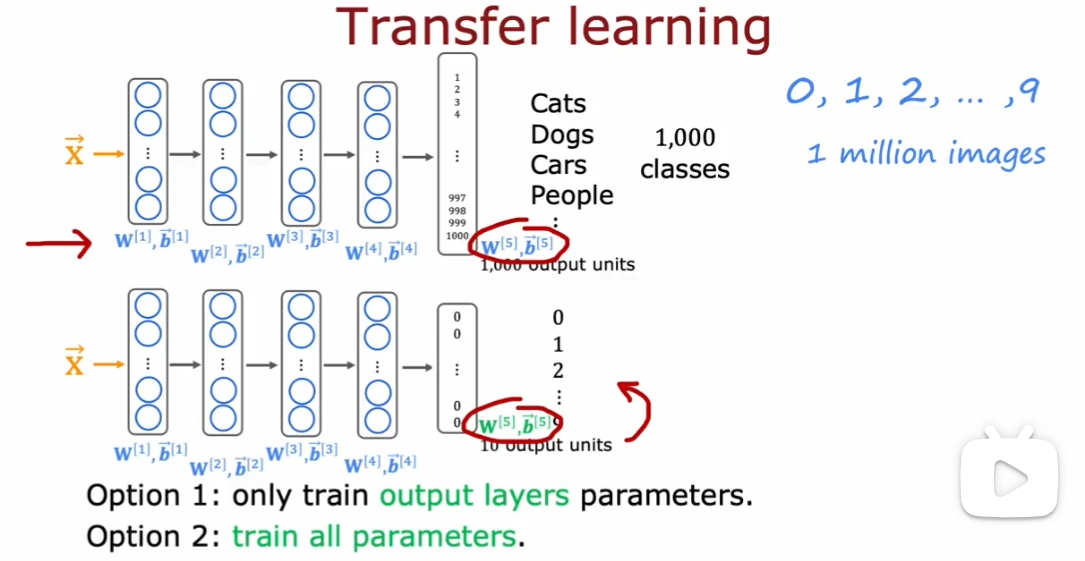• 先在大型数据集上进行训练，然后在较小的数据集上进一步参数调优，这两个步骤称为监督预训练（supervised pretraining）
• 然后运行梯度下降等算法在新数据集上，进行微调（fine tuning）

## 4.4. 构建 ml 系统的周期

1. 确定项目范围
2. 收集数据
3. 训练模型
4. 部署模型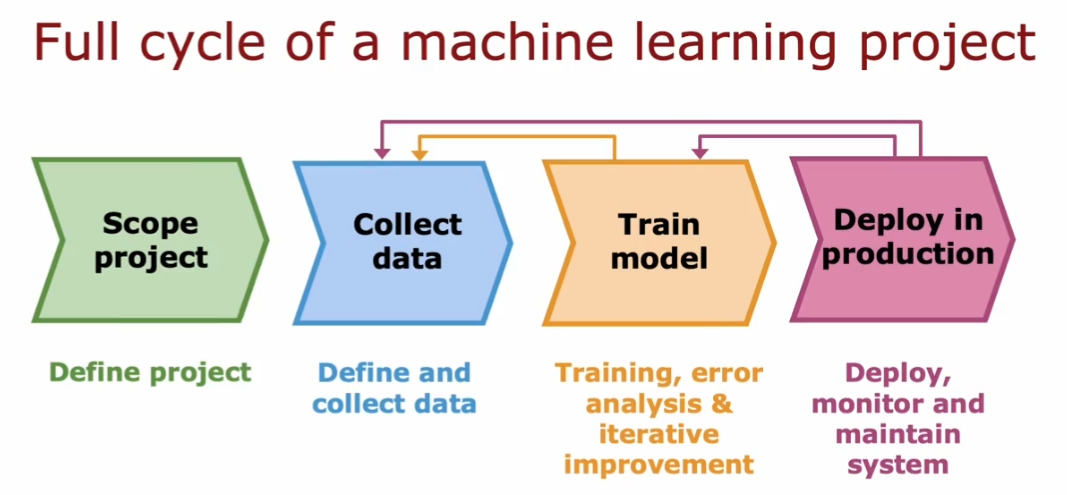### 4.4.1. MLOps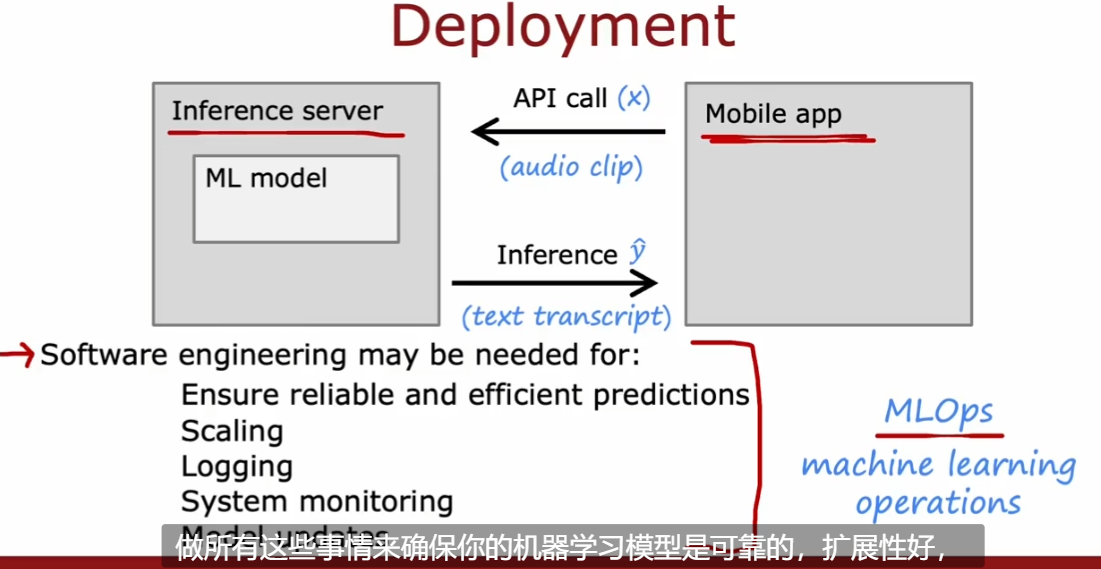• 有点类似 DevOps

## 4.5. 数据倾斜

• 评价一个罕见类的学习算法性能
• 构造混淆矩阵（2 * 2 矩阵）
• 精度（Precision）：评价算法是否准确：当算法诊断有病时，确诊的概率
• 召回率（Recall）：评价算法对真正病人是否有用（样本中的正例有多少被预测正确了或找的全）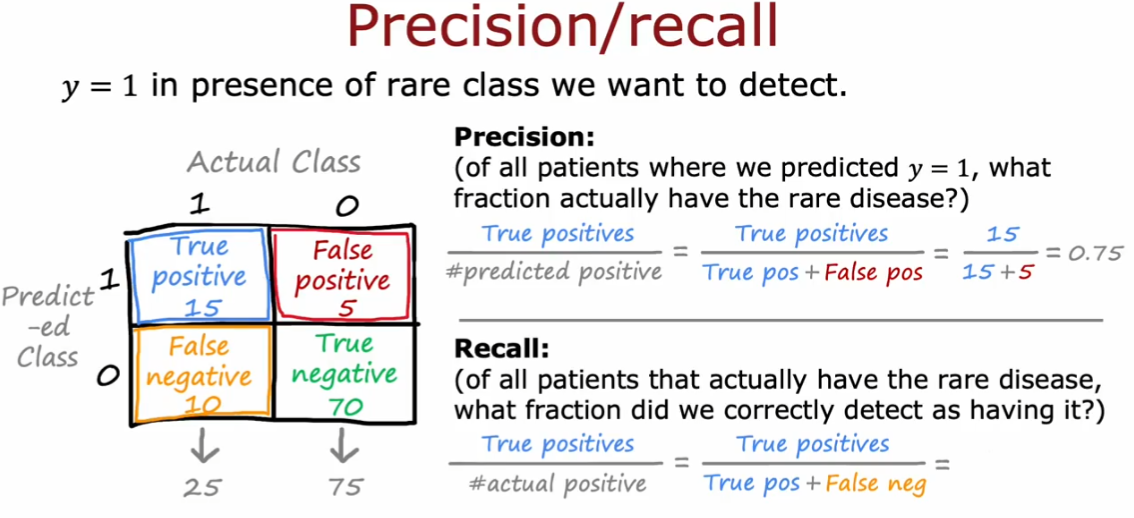### 4.5.1. Trade off between P and R

• 建议根据具体的应用场景进行权衡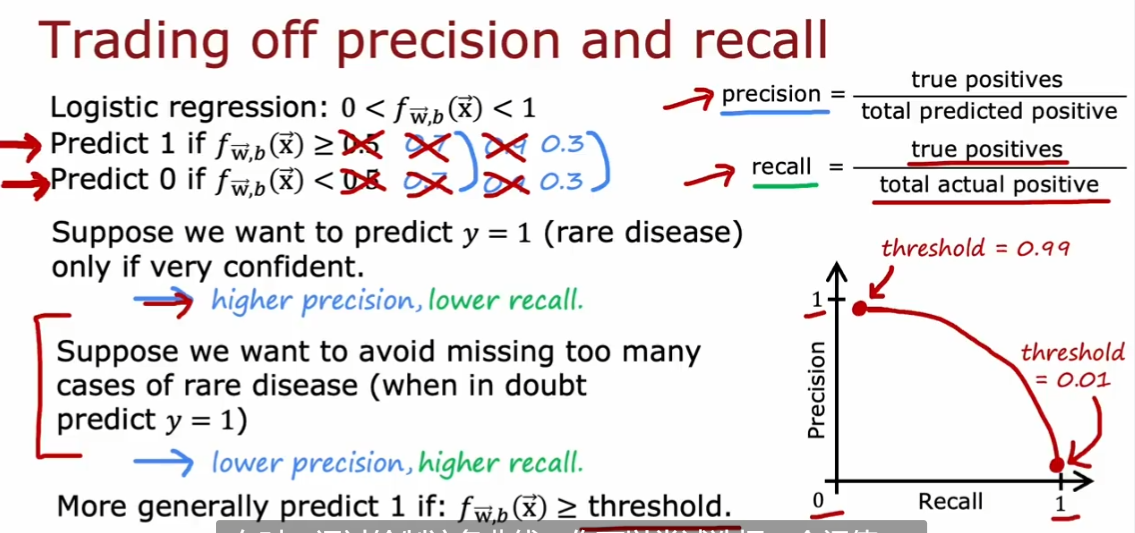#### 4.5.1.1. F score

• 权衡 Recall 和 Precision 的参数

$$F_1 \ scrore = \frac{1}{\frac{1}{2}(\frac{1}{P} + \frac{1}{R})} = 2\frac{PR}{P+R}$$

• 这个式子会关注二者中较小的部分（其实就是 P 和 R 的调和平均值）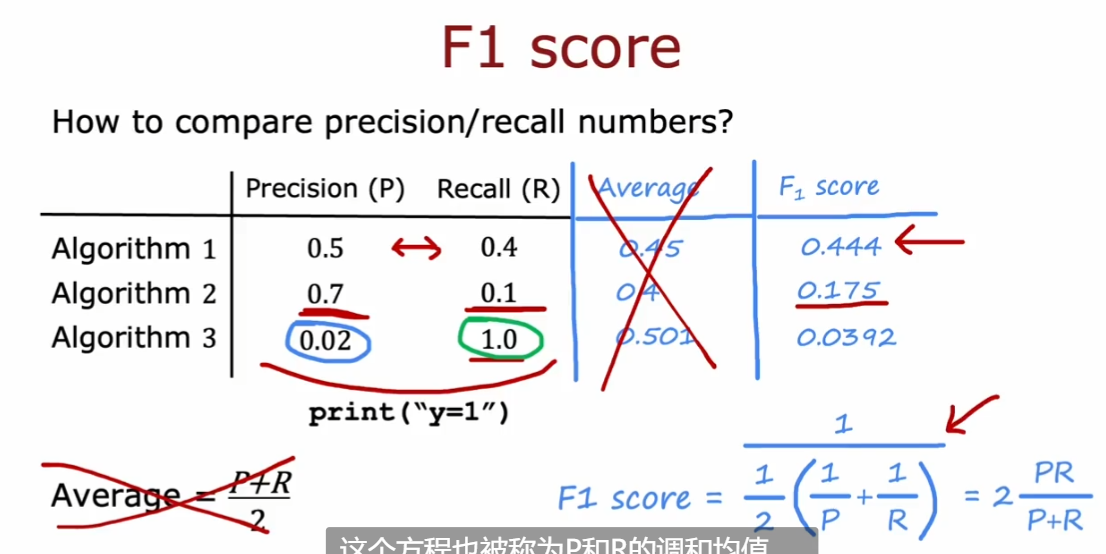## 4.6. 决策树

• Decision Tree

### 4.6.1. 步骤

• 选择根节点的特征
• 选择在每个节点上区分的特征（为了最大化的分类，保证分类纯度 purity）
• 何时停止树的划分（stop splitting）
• 当结点里 100%都是同一类
• 当分裂（split）结点会导致树超过最大深度
• 当分裂（split）后对纯度产生的增益小于阈值（threshold）
• 当结点里 examples 的数量小于阈值（threshold）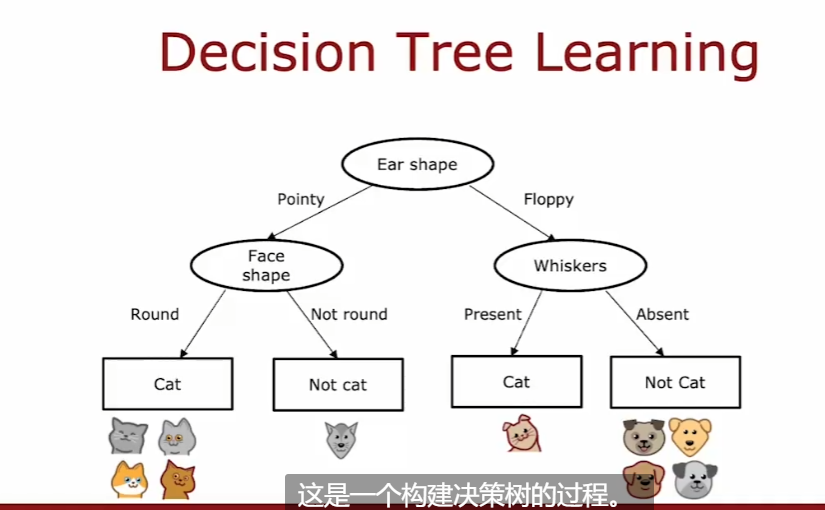### 4.6.2. 熵

• entropy
• 度量样本不纯程度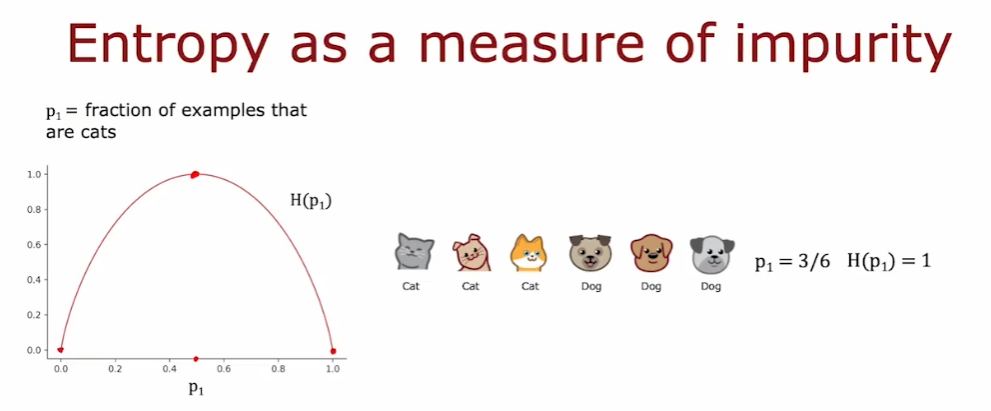$$p_0 \ = \ 1\ - \ p_1 \ H(p_1) = -p_1log_2(p_1) - p_0log_2(p_0) \ \ \ \ \ \ \ \ \ \ \ \ \ \ \ \ \ \ \ \ \ \ \ \ \ \ \ \ \ = -p_1log_2(p_1) - (1-p_1)log_2(1-P_1) \ Note: 0log(0) = 0$$

#### 4.6.2.1. 如何利用熵度量 node

• 使用加权平均。因为我们认为分到了较多 examples 的分支的熵是更重要的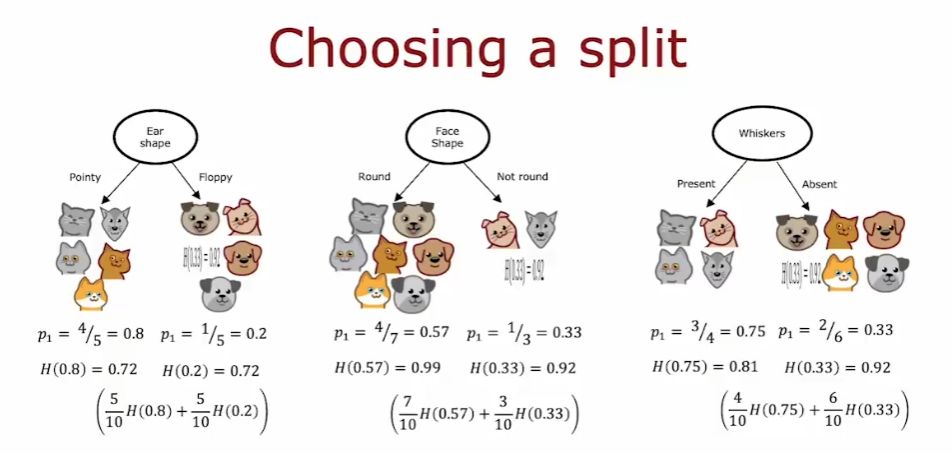#### 4.6.2.2. 信息增益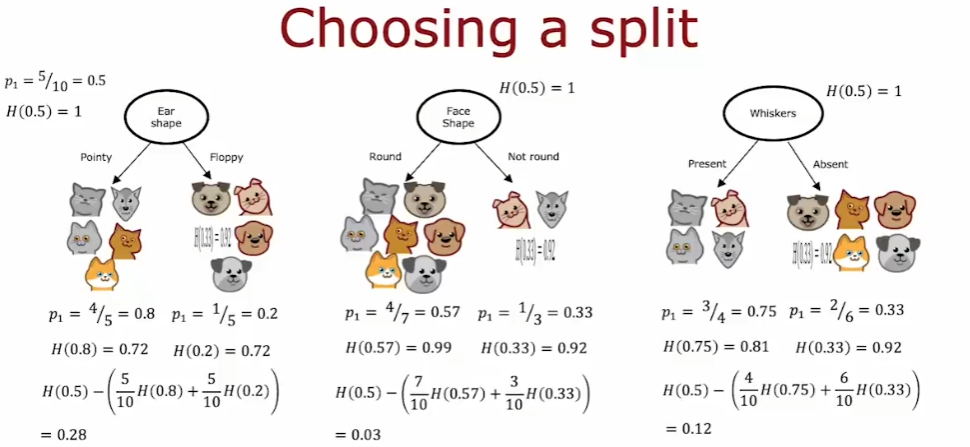• 计算公式### 4.6.4. Decision Tree Learning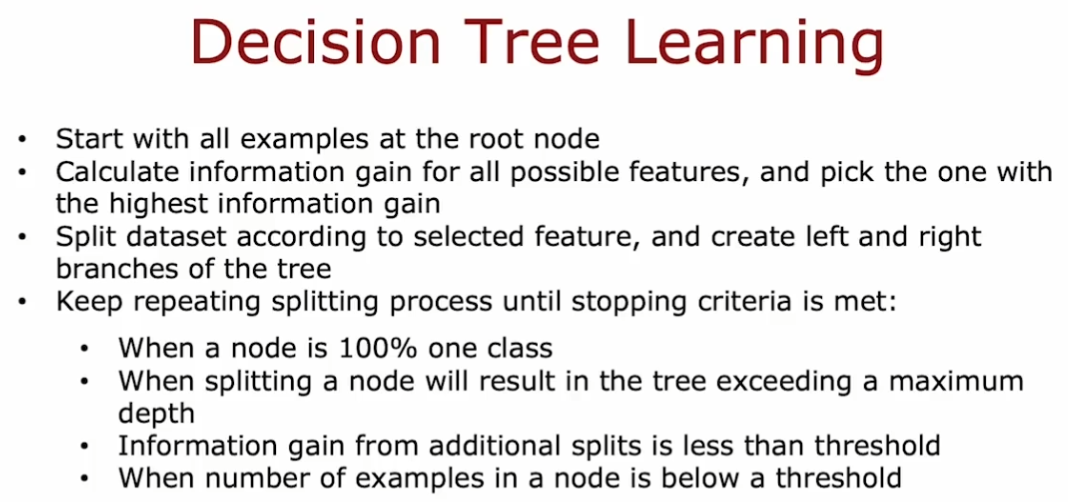• building decision tree 是一个递归过程

### 4.6.5. one-hot 编码

• 如果一个分类特征有 k 种可能的取值，那么把这 k 种特征按照 0、1 进行编码取值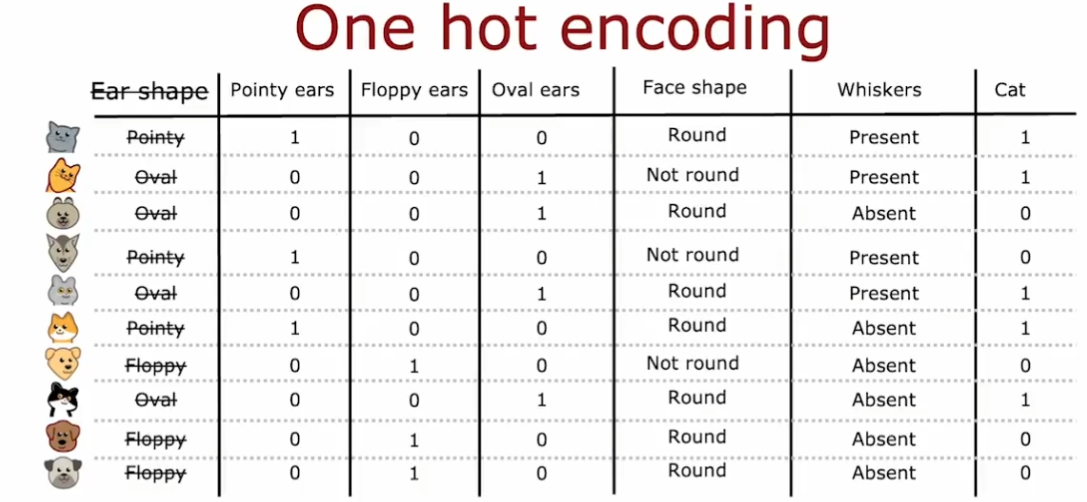• 如果看任何一行，总有一个特征是 1，因此称之为 one-hot（独热）编码

• one-hot 编码不仅适用于决策树，还适用于神经网络

### 4.6.6. 处理连续变量

• 对连续变量进行划分处理（分类），即将连续变量区间设置阈值，将其分为 2 个子集

• 分类的标准是谁贡献了更大的信息增益

• 一般划分值的候选方法是：将值 list 排序，然后取所有值之间的中点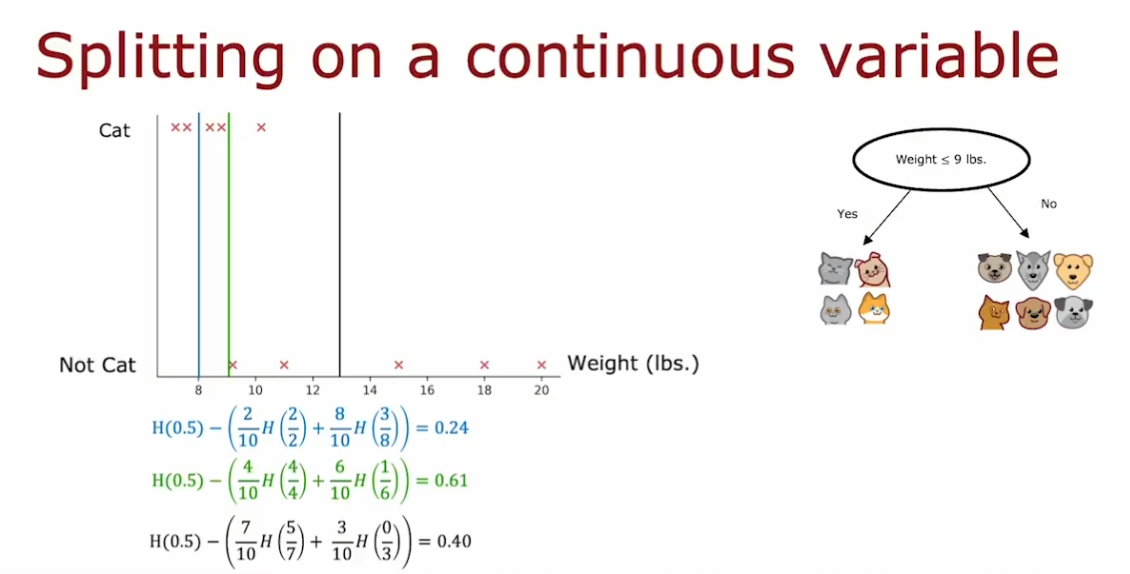## 4.7. 回归预测中的决策树

• 决策树会根据叶子结点中样本数据的平均值做出预测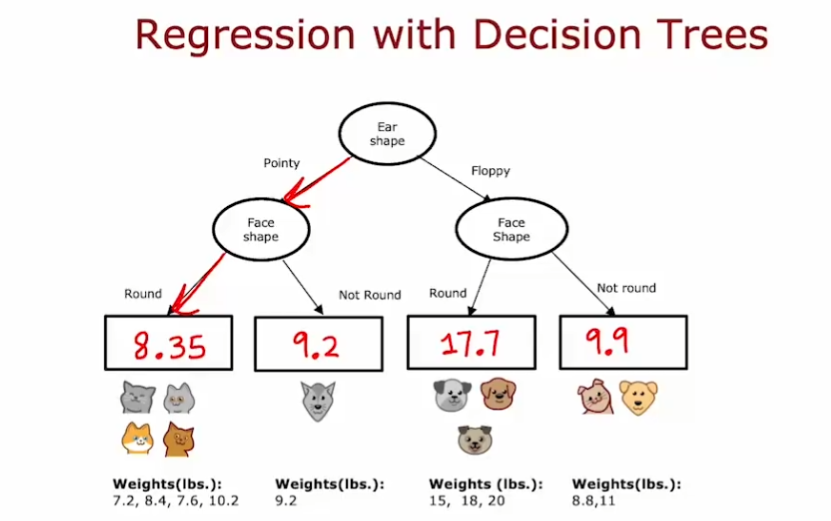• 在回归预测中，决定结点选择哪种划分策略的标准不再是熵，而是带权方差（variance）

• 就像最终决策树的衡量公式是信息增益，我们在这里计算的也实际上是方差减少量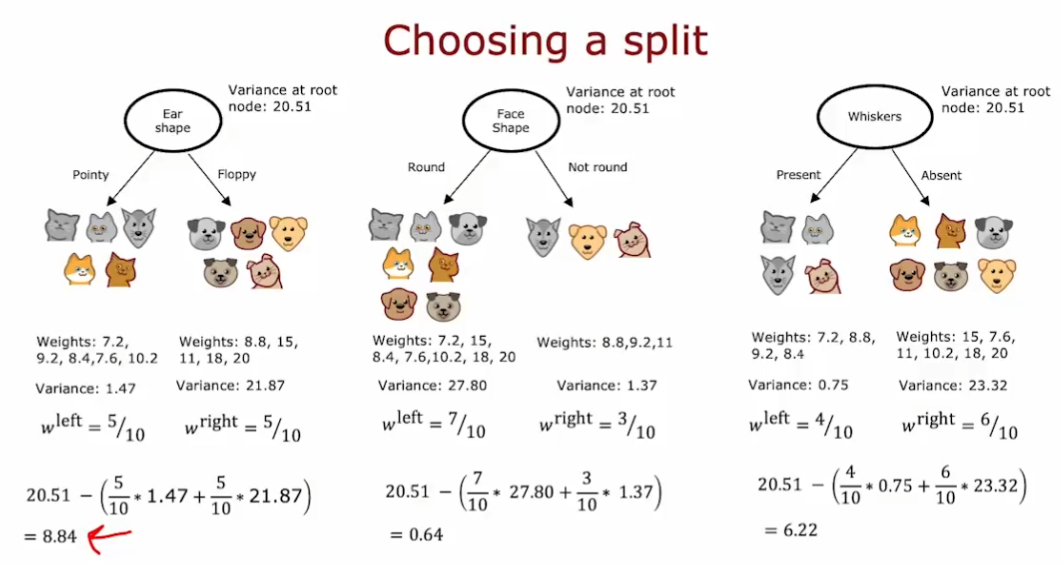## 4.8. 决策树集合

• 使用单个决策树的缺点是：其对数据的微小变化非常敏感。(一旦数据微小变化，那么结点的信息增益可能会变化很多，从而导致决策树变的完全不同)

• 解决方法：构建更多的决策树，即决策树集合，使得预测结果更健壮
• 使用树集合，然后都运行数据，进行预测。多数的结果即为预测结果。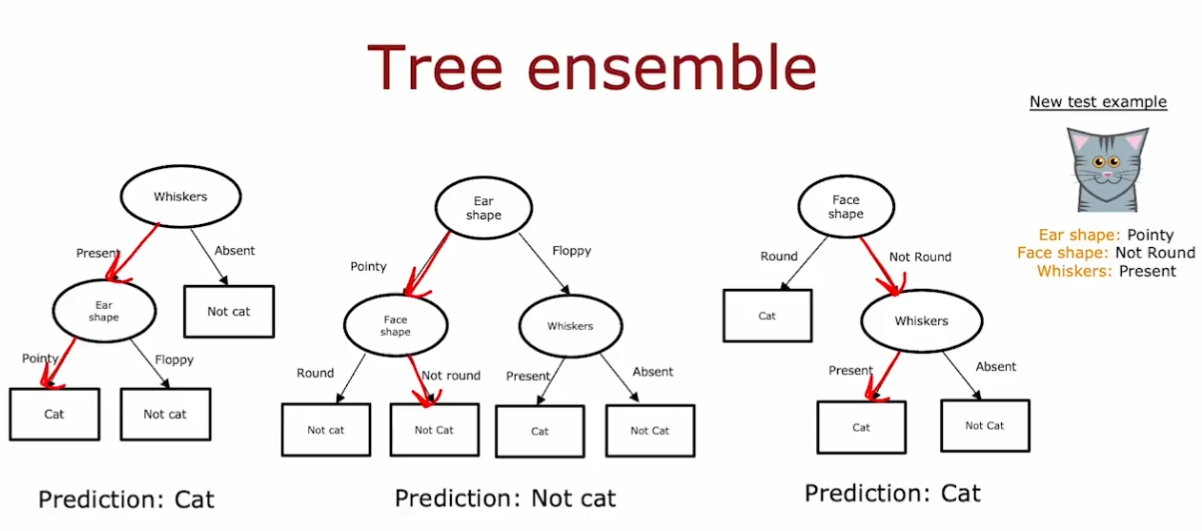### 4.8.1. 有放回抽样

• Sampling with replacement

### 4.8.2. 随机森林算法

#### 4.8.2.1. 袋装决策树算法

• 对于一个大小为 m 的训练集
• 使用有放回抽样获得同样大小为 m 的训练集，然后训练出一个决策树
• 重复以上过程直到训练出需要的决策树数量（一般是 100，记为**B**）
• 问题
• 根节点及其附近的特征仍然相似
• 导致算法不够精确，需要改进

#### 4.8.2.2. 改进成随机森林算法

• 在每个结点中，当需要选择一个特征来 split node 时，如果有 n 个特征可以选择，那么我们每次随机选择k（k < n ）个特征构成 n 个特征的子集，让算法在这个子集中进行选择（即计算信息增益然后进行 split）
• 比单一决策树更健壮的原因是：随机森林算法探索了更多训练集微小变化的可能，能够对有放回取样过程导致的所有数据变化进行平均，使得训练出来的模型更 robust

### 4.8.3. XGBoost 增强决策树算法

• 非常类似于针对性训练，比如刻意训练不熟练的一段，而不是总是训练整首曲子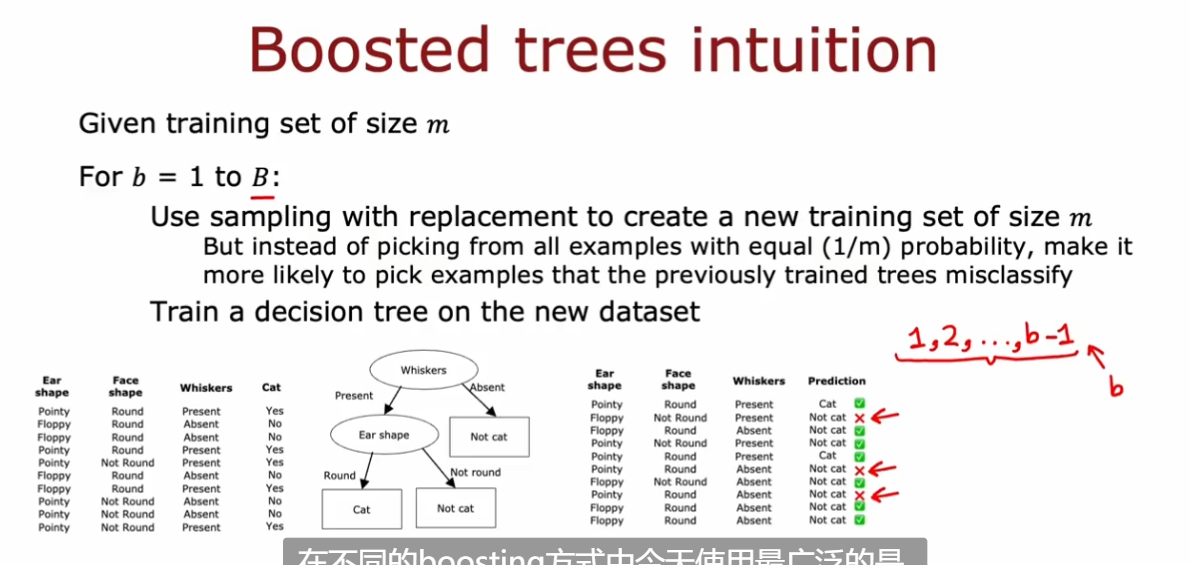• 增强决策树的一种开源实现
• 高效
• 对默认拆分条件有很好的选择
• 内置正则化以防止过拟合
• 和 dl 一样，是算法竞赛的热门算法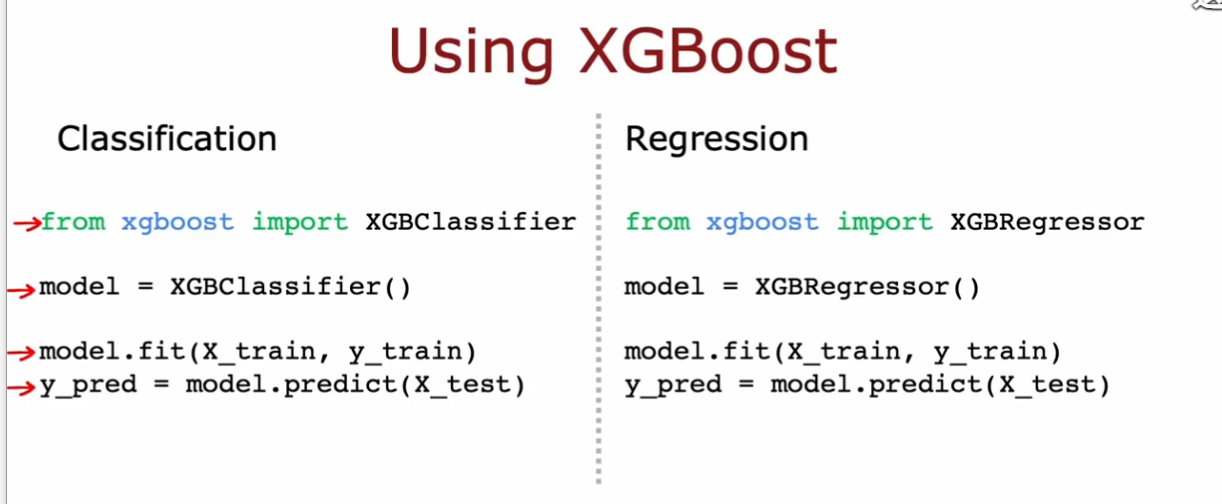## 4.9. 决策树和神经网络的使用时机

• 决策树和树集合
• 对表格化（结构化）tabular（structured）数据表现很好
• 不建议在非结构化数据上使用（图像、音频、文本）
• 训练迅速
• 小的决策树可能是人类可以理解的（可解释性）
• 神经网络
• 对结构化和非结构化数据都表现良好
• 比决策树慢（训练时长等）
• 可以使用迁移学习（而决策树不行）
• 当构建多个模型共同协作的系统时，神经网络模型更容易串在一起（决策树一次只能训练一个）